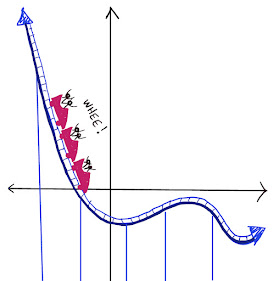## Wednesday, November 11, 2015

### Geometric Progressions

For the solution, click "Read More" below.

There are different ways to reason through these problems. including by using the formula for a geometric series. Here are some ideas for reasoning through the problems without using the formula.

Problem #1
One-third of the square is shaded.

For this square we can focus one quarter since they differ only by a rotation.

Notice that we can think of this piece as a series of L-shaped pieces that get smaller and smaller. While the L's are all different in size, they each have the same fraction shaded.

Since 1/3 of each "L" is shaded, then 1/3 of the whole square is shaded.

Problem #2

Two-thirds of the square is shaded.

Notice that two-fourths are shaded completely, and then 1/3 of the other two-fourths are each 1/3 shaded for the same reason explained above.  Adding the parts we get 1/2 + (1/3 x 1/2) = 2/3

Problem #3

Two-thirds of the square is shaded.

Notice that we can break down the square into Ls where each one is 2/3 shaded:

So the whole thing is 2/3 shaded.

Problem #4

The square is half shaded.  Notice that for every triangle that is shaded, there is a corresponding triangle that is not shaded.  Since the two parts are equal, it is half shaded.

Problem #5

Each quarter differs only by a rotation, so let's focus on one quarter.

This square, like some of the others, is made of nested Ls.  The largest one, which is 3/4 of the square, is 2/3 shaded.

The others, which together form 1/4 of the square, are all together 1/3 shaded, because of reasoning given above in problem 1.

All together we get (2/3 x 3/4) + (1/3 x 1/4) = 7/12.